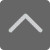爱智康资讯站

课程咨询: 4000-121-121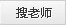北京小学一年级数学下册1单元检测题

2019-04-12 17:53:26 　来源：网络整理

北京小学一年级数学下册1单元检测题小学数学学习的重视是我们的基础知识，大家一定要掌握科学的学习方法，提高数学的学习效率。下面就是小编为大家带来的北京小学一年级数学下册1单元检测题，希望可以帮助到大家。

想要了解【小学数学试题】的相关资料，请点击加入【爱智康小学交流福利群】，并直接向管理员“小康康”索取！爱智康小学交流福利群会不定期免费发放学习资料，小学政策等相关消息，请持续关注！

一、口算。

13-9= 　　16-7=　　 12-3=　　 11-8= 　　17-9=　　 18-9= 　　15-8=

16-9= 　　14-5=　　 15-6=　　 11-7= 　　14-7= 　　12-5=　　 13-4=

11-2= 　　12-6= 　　11-9=　　 13-8=　　 11-3= 　　14-9= 　　15-7=

11-4= 　　15-5= 　　5+6=　　 4+9= 　　3+8= 　　11-5=　　 11-6=

12-4=　　 12-7= 　　12-8=　　 12-9= 　　13-5= 　　13-6= 　　13-7=

14-6=　　 14-8= 　　15-9= 　　16-8=　　 17-8= 　　4+7= 　　8+5=

14-9-2=　　 12-7+6= 　　7+6-8= 　　6+3+4= 　　11-9+8= 　　8+8-7=

二、在( )内填上“>”“<”或“=”。(6分)

15-8( )10 　　11-3( )11-4　　 18-9( )12-2

13-9( )5 　　15-10( )14-9 　　13-7( )13-6

小编推荐：

以上就是小编特意为大家整理的北京小学一年级数学下册1单元检测题希望对有需要的同学提供帮助，大家如果在学习中有什么疑问，欢迎拨打爱智康免费电话：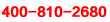!那里有专业的老师为大家解答。

2019年精品学习包：

•小学语|数|英

历年试卷、北京30所校练习题、名著|重点校巡礼|等100讲视频、纸质书籍领取

•初中九大学科

历年真题、期末|期中|志愿填报|重点校巡礼|等100讲视频、纸质书籍领取

•高中九大学科

历年真题、期末|期中|志愿填报|重点校巡礼|等100讲视频、纸质书籍领取

•更新中

学习包内容持续更新中......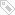标签：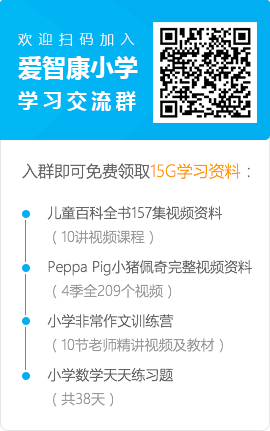• 全国课程在线咨询
• 咨询热线：4000-121-121
• 扫描注册有礼

• 让学习更有效